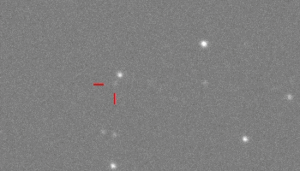# Asteroid Size Estimation

Quote of the Day

The job of a successful leader is to build relationships that are based on mutual respect and the recognition that others know things that we may need to know to get the job done.

— Edgar Schein. This is as good a description of leadership that I have found.

## IntroductionFigure 1: View of 2012 TC4 From Earth. Note how small and faint the asteroid is in the photo. (Source)

I often see announcements of Near-Earth Objects (NEOs) in the scientific press. For asteroids, these announcements are usually accompanied by a size estimate of the asteroid. In this post, I will discuss a commonly used formula for the effective spherical diameter of an asteroid based on its normalized brightness (i.e. absolute magnitude).

Most asteroids are such small objects that astronomers cannot obtain a large enough image to make a direct measurement. Instead, the astronomers estimate the size of the asteroid by on its range and brightness. Unfortunately, the brightness of an asteroid depends on it surface composition, which is usually unknown when the asteroid is little more than a point of light when first seen from Earth. Astronomers are then forced to give a wide range of possible diameters to account for their surface composition uncertainty.

## Background

### Definitions

Apparent Magnitude (V)
The visual brightness of an asteroid when observed and measured visually or with a CCD camera. (Source)
Absolute Magnitude (H)
The visual magnitude of an asteroid if it were 1 AU from the Earth and 1 AU from the Sun and fully illuminated, i.e. at zero phase angle – a geometrically impossible situation. (Source)
Geometric Albedo (pV)
The ratio of the brightness of a planetary body, as viewed from the Sun, to that of a white, diffusely reflecting sphere of the same size and at the same distance. Zero for a perfect absorber and 1 for a perfect reflector. (Source)
I smiled when I read this description of geometric albedo – it is VERY similar to the description of target strength as used by the sonar community. I am always amazed at the similarity between the various engineering and scientific disciplines.
Bond Albedo (A)
The Bond albedo is the fraction of power in the total electromagnetic radiation incident on an astronomical body that is scattered back out into space. The Bond albedo is related to the geometric albedo by the expression $latex A=p\cdot q&s=1$. , where q is termed the phase integral. (Source)
Equivalent Spherical Diameter
The equivalent spherical diameter of an irregularly shaped object is the diameter of a sphere of equivalent volume. (Source)

### Key Formula

Equation 1 shows the formula that I see being used in a number of papers.

 Eq. 1 $latex \displaystyle D({{p}_{V}},H)=\frac{{1329}}{{\sqrt{{{{p}_{V}}}}}}\cdot {{10}^{{-0.2\cdot H}}}\left[ {\text{km}} \right]&s=2$

where

• D is the diameter of the asteroid [km].
• pV is geometric albedo [dimensionless].
• H is absolute magnitude of the asteroid [dimensionless].

The detailed derivation of Equation 1 is a bit involved, but quite interesting. See this document for details (section 4.2).

### Post Objective

This post will consist of two calculations using Equation 1:

## Analysis

### Setup

Figure 2 shows my setup for the calculations.

### Two Asteroid Examples

Figure 3 shows my calculations for the effective diameter of 2012 TC4 and Ceres. My  calculations are in good agreement with the published information.

### Minor Planets Center Table

Figure 4 shows my calculations for duplicating the effective diameter versus absolute magnitude table. Within the rounding used by the Minor Planets Center, I duplicated their results.

## Conclusion

I was able to use Equation 1 to duplicate a number of results for asteroid sizes. This exercise showed me that it is difficult to get an accurate size estimate for asteroid because their albedos can vary so widely.

Save

Save

Save

Save

Save

Save

Save

Save

This entry was posted in Astronomy. Bookmark the permalink.